### Hyperparameter Optimization of Long-Short Term Memory using Symbiotic Organism Search for Stock Prediction

1,2Computer Science Department, Bina Nusantara University, Jakarta, Indonesia.

Abstract

Producing the best possible predictive result from long-short term memory (LSTM) requires hyperparameters to be tuned by a data scientist or researcher. A metaheuristic algorithm was used to optimize hyperparameter tuning and reduce the computational complexity to improve the manual process. Symbiotic organism search (SOS), which was introduced in 2014, is an algorithm that simulates the symbiotic interactions that organisms use to survive in an ecosystem. SOS offers an advantage over other metaheuristic algorithms in that it has fewer parameters, allowing it to avoid parameter determination errors and produce suboptimal solutions. SOS was used to optimize hyperparameter tuning in LSTM for stock prediction. The stock prices were time-series data, and LSTM has proven to be a popular method for time-series forecasting. This research employed the Indonesia composite index dataset and assessed it using root mean square error (RMSE) as a key indicator and the fitness function for the metaheuristic approach. Genetic algorithm (GA) and particle swarm optimization (PSO) were used as benchmarking algorithms in this research. The hybrid SOS-LSTM model outperformed GA-LSTM and PSO-LSTM with an RMSE of 78.799, compared to the GA-LSTM model with an RMSE of 142.663 and the PSO-LSTM model with an RMSE of 529.170.

Keywords:Long-short term memory, Metaheuristic algorithm, Symbiotic organism search, Genetic algorithm, Particle swarm optimization, Optimization, Hyperparameters tuning, Stock prediction.

# 1. Introduction

Stock prediction is one of the most important aspects of limiting risk in the capital market, specifically in transactional operations . Stock prediction research is continually being conducted to aid in risk management when buying and selling stocks. Time-series data on stock prices is commonly used for this purpose. Time-series data may be predicted using a variety of approaches, including Autoregressive Integrated Moving Average (ARIMA), Support Vector Regression (SVR), and Long-Short Term Memory. Numerous studies have shown that LSTM outperforms SVR and ARIMA [2, 3]. Despite its favorable outcome in certain studies, one of the obstacles that might impact prediction results in LSTM is hyperparameter tuning . Machine learning requires parameters to be modified by a data scientist or researcher to produce the best prediction results. These techniques, known as hyperparameter tuning and hyperparameter optimization in deep learning, have a significant influence on predictive results . The number of hidden layers, the number of neurons, the learning rate, the dropout rate, and the type of optimizers are all factors to consider. Deep learning architecture with several hidden layers provides higher generalization than single-layer architecture. The number of hidden layers assigned in deep learning affects the delivery of effective predictive results . However, it is challenging to select the optimal number of neurons in deep learning layers. If the number of neurons in a recurrent neural network, such as Long-Short Term Memory (LSTM), is too small, LSTM will be unable to recall all the information required to create an optimum prediction. On the other hand, a large number of neurons may result in an overfitting model . To obtain optimal prediction outcomes, another key hyperparameter is the learning rate. This is a set of hyperparameters that allow the researcher to regulate the pace of change in deep learning weight. Finally, using varying dropout levels in the LSTM model might improve its performance and generalization.

One way to optimize hyperparameter tuning in LSTM is to integrate the LSTM model and the metaheuristic algorithm. Symbiotic Organism Search (SOS), which was introduced in 2014, is one of the metaheuristics methods. It is an algorithm that replicates the symbiotic relationships that organisms employ to thrive in their environment . This method is commonly used in civil and construction areas to address engineering obstacles. SOS offers benefits over other metaheuristic algorithms in that it has fewer parameters, allowing it to avoid parameter determination mistakes, which produce suboptimal solutions . SOS has been proven to outperform other metaheuristics, such as Particle Swarm Optimization (PSO) and Genetic Algorithm (GA), where the algorithm was used to optimize weights and biases on Feedforward Neural Networks (FNNs) . Although SOS has been used in machine learning, most researchers have used this algorithm to optimize weight and biases and have not used it for hyperparameter optimization.

Previous studies relating to this topic may be classified into two categories: LSTM research and improving hyperparameter tuning in deep learning using metaheuristic algorithms. Table 1 summarizes all related works on LSTM research and improving hyperparameter tuning in deep learning that have been reviewed in this paper.

Table 1.Summary related works.
 Ref. Dataset Method Result Abbasimehr, et al.  Demand Optimized LSTM ETS ARIMA SVM ANN Optimized LSTM achieved the best performance with an RMSE of around 2595.96 and SMAPE around 0.1085 compared to other methods and unoptimized LSTM. Abdual-Salam, et al.  Stock Price DE-ANN PSO-ANN DE-ANN has the highest score on RMSE, MSE, and MAE Chniti, et al.  E-commerce LSTM SVR LSTM acquires an RMSE of around 23.640, smaller than SVR with an RMSE of 39.912. Chung and Shin  Stock Price GA-LSTM MSE is around 181.99, MAE is around 10.21, and MAPE is around 0.91%. Girsang, et al.  Stock Price SE-LSTM LSTM ARIMA SE-LSTM model is better than unoptimized LSTM and ARIMA with RMSE around 538.914, MAE around 402.977, MAPE around 1.437%, and an R2 score of around 0.961. Göçken, et al.  Stock Price HS-ANN GA-ANN The average stock prediction result for HS-ANN has a better result than GA-ANN, with a MAPE value of 3.38. McNally, et al.  Bitcoin LSTM RNN ARIMA LSTM and RNN acquire better RMSE and accuracy than ARIMA. Nanda and Jonwal  Channel Equalizer WNN-SOS WNN-CSO WNN-CLONAL WNN-SOS has the highest MSE and BER compared to other algorithms. Siami-Namini, et al.  Bitcoin LSTM ARIMA LSTM acquires an RMSE of around 64.213, which is better than ARIMA with an RMSE of around 511.481. Qing and Niu  Weather Persistence LR BPNN LSTM LSTM achieves a better RMSE than Persistence, LR, and BPNN. Wu, et al.  UCI Machine Learning Repository SOS-FNN PSO-FNN GA-FNN CS-FNN BBO-FNN SOS-FNN has better MSE results than PSO-FNN, GA-FNN, CS-FNN, and BBO-FNN.

1.1. Studies on Long-Short Term Memory (LSTM)

There has been a huge amount of research on the Long Short-Term Memory (LSTM) algorithm, which is used to forecast time-series data. According to various study findings, LSTM outperforms other algorithms, such as Support Vector Regression (SVR), Autoregressive Integrated Moving Average (ARIMA), and Linear Regression (LR). In the EUROPEAN market, LSTM was used to estimate telephone pricing through E-commerce . In this study, a comparison between LSTM and SVR to forecast time-series data yielded an RMSE value of 39.912 for SVR and an RMSE value of 23.640 for LSTM. To forecast bitcoin prices, LSTM, Recurrent Neural Network (RNN), and Autoregressive Integrated Moving Average (ARIMA) were compared . In this study, RNN and LSTM were shown to be effective models for predicting time-series data and provided a substantially lower RMSE value than ARIMA. Another study comparing LSTM and ARIMA to forecast bitcoin prices found that LSTME had a lower RMSE (64.213) than ARIMA, which had an RMSE of 511.481 . A study of a weather prediction dataset compared LSTM to the Persistence method, Linear Regression (LR), and Backpropagation Neural Network (BPNN) . Utilizing the weather forecast dataset supplied by LSTM, the RMSE value is less than those of Persistence, LR, and BPNN in numerous rounds of the experiment. Finally, a study comparing optimized LSTM, Error Trend Seasonal (ETS), ARIMA, Support Vector Machine (SVM), and Artificial Neural Network (ANN) for demand forecasting found that optimized LSTM outperformed other methods in terms of both RMSE and SMAPE .

1.2. Studies on Optimizing Hyperparameter Tuning in Deep Learning using Metaheuristic Algorithms

Various scholars have researched the optimization of hyperparameter tuning for deep learning using metaheuristic algorithms. One of the most extensively used metaheuristic algorithms is the genetic algorithm. Research on the hybrid model LSTM and the genetic algorithm for stock market prediction has shown that it outperforms the benchmarking model . To forecast time-series data, a hybrid method was utilized on the LSTM network, which was merged with a genetic algorithm to identify modified time frames and the number of LSTM units. This analysis revealed that the hybrid model of LSTM and genetic algorithm (LSTM-GA) outperformed the benchmark model, with MSE around 181.99, MAE around 10.21, and MAPE around 0.91 percent.

Differential Evolution (DE) and Particle Swarm Optimization (PSO) were utilized to optimize an Artificial Neural Network (ANN) for stock market prediction, and the results were encouraging . The ANN model that did not employ optimization methods performed better than the two metaheuristic algorithms. A hybrid model was employed to forecast the stock market performance of seven firms chosen by researchers. DE algorithms proved faster and more accurate than PSO algorithms. With the parameters RMSE, MSE, and MAE, DE-ANN outperformed PSO-ANN.

Search economics is another metaheuristic technique used for hyperparameter optimization in deep learning. In 2015, a new metaheuristic algorithm called search economics was introduced. Search economics is intended to reduce the computing time required to optimize a system and has been shown to outperform the Genetic Algorithm in WSN deployment . This algorithm has been used to optimize LSTM for stock market prediction and compare it to the benchmark model . The hybrid model SE-LSTM was compared to LSTM and ARIMA, and it was determined that it might improve stock prediction outcomes. With RMSE around 538.914, MAE around 402.977, MAPE around 1.437 percent, and an R2 score of approximately 0.961, the SE-LSTM model outperformed the unoptimized LSTM and ARIMA.

Harmonic search (HS) is a metaheuristic method used to improve an Artificial Neural Network (ANN) and has been compared to the Genetic Algorithm (GA) for stock market prediction . HS-ANN displayed a higher average stock prediction outcome than GA-ANN, with a MAPE value of 3.38.

In certain studies, Symbiotic Organism Search (SOS) has been utilized to optimize weights and biases in deep learning rather than hyperparameter tuning. SOS is used to train Feedforward Neural Networks (FNN) more effectively by refining the deep learning algorithm's weights and biases . SOS has been shown to outperform many metaheuristic algorithms, including Cuckoo Search (CS), Particle Swarm Optimization (PSO), Genetic Algorithm (GA), Multiverse Optimizer (MVO), Gravitational Search Algorithm (GSA), and Biogeography Based Optimizer (BBO), in terms of optimizing weights and biases. Another study used SOS to train weights and biases on a Wavelet Neural Network (WNN) to create an equalizer to reduce inter-symbol interference on communication channels . In this study, the Cat Swarm Optimization (CSO), Clonal Selection Algorithm (CLONAL), and Least Mean Square Algorithm (LMSA) were compared. The results showed that WNN-SOS generates an equalizer design that is resistant to burst fault circumstances.

# 2. Materials and Methods

The hybrid method SOS-LSTM will be utilized to optimize hyperparameter tuning. As Figure 1 illustrates, this study is separated into five processes: data collection, data pre-processing, parameter initialization, modeling with SOS-LSTM, and assessment. Stock price data from the Indonesian Stock Exchange is used in this study. Following the data collecting procedure, the dataset needs are pre-processed.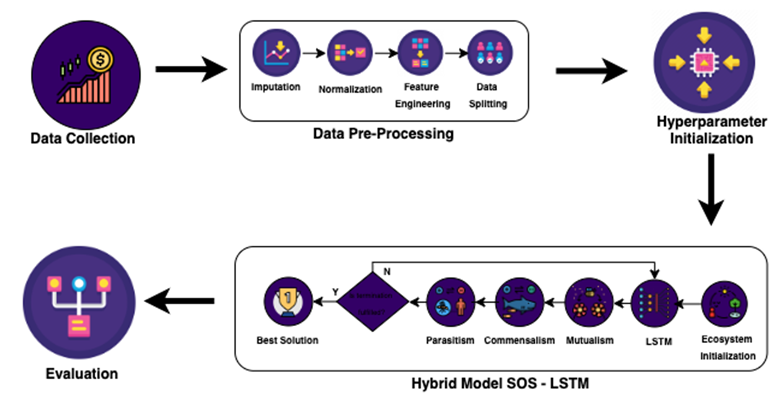Figure 1.Research methodology.

2.1. Data Collection

The dataset utilized in this study comprises the stock price data of an Indonesian financial institution and was taken from Yahoo Finance. The stock price data we used spans daily records from 1990 to 2021 and includes four types of stock prices: opening, closing, highest, and lowest price. The closing price will be utilized to forecast the target price.Figure 2. Data pre-processing.

2.2. Data Pre-Processing

As shown in Figure 2, the first stage to be completed is the imputation. Imputation is a method for filling empty or null values in data. Backward filling will be used as the imputation strategy in this study. The next valid observation will be utilized to fill the gap or to return null data. Because the dataset utilized in this study is time-series data, any vacant stock price data will be replaced with following-day stock price data.

Normalization is the next step in the pre-processing stage. The objective of data normalization is to remove anomalies or outliers from the data while also minimizing variances in the data. The normalization procedure is required for time-series data, such as stock prices, to reduce the model's reliance on changes in very significant data variations. The MinMax Scaler will be used to normalize the data in this study.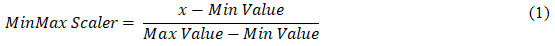As indicated in Equation 1, x represents the value to be normalized, MinValue represents the minimum value of the feature to be normalized, and MaxValue represents the highest value of the feature to be normalized.

Following normalization, the data will be processed for feature engineering. This is one of the most important responsibilities when preparing data for the machine learning process. In this method, we create relevant new features based on the characteristics supplied to increase the performance of a machine learning model . For instance, building a sliding window feature is one type of feature engineering commonly used by data scientists to analyze time-series data and improve the performance of a machine learning model .

The final stage of pre-processing is to partition the dataset into training, validation, and testing data. A machine learning model is trained and created using the training dataset. In each step, the validation dataset is utilized to assess hyperparameter tweaking. The test dataset is used to compare the performance of the hybrid models GA-LSTM, PSO-LSTM, and SOS-LSTM and to select the most suitable method. The objective of partitioning the dataset is to avoid model overfitting and boost the machine learning model's dependability and resilience. In this study, 80 percent of the dataset will be utilized as training data,10 percent as validation data, and 10 percent as testing data.

2.3. Hyperparameter Initialization

The parameter initialization method is designed to apply an initial value to the LSTM machine learning model. SOS, GA, and PSO algorithms will be utilized to optimize these hyperparameters. The hyperparameters that will be employed are:

a) The number of neurons: The number of neurons chosen for each layer of the LSTM network is a difficult issue. If the number of neurons is too small, the LSTM will be unable to recall all the information required to generate optimal predictions. On the other hand, if the number of neurons is too great, LSTM will overfit the training data .
b) The number of hidden layers: The number of hidden layers demonstrates that the deep learning neural network design is more general than one with single-layer architecture [6, 20]. Therefore, it is critical to verify the model's performance using more than one layer.
c) Dropout rate: Implementing a varied dropout rate might improve the LSTM model's performance by enhancing the model's generalization .
d) Learning rate: The learning rate is a hyperparameter that modifies the model's weighting and biases. Choosing an appropriate learning rate is critical to achieving optimal model performance as it affects the weighting and biases of the model .
e) Type of optimizers: In a neural network, the optimizer is in charge of minimizing an objective function. Model performance may be improved by combining optimizer and learning rate .
f) The number of epochs: When training a machine learning model, the number of epochs must be specified to avoid underfitting or overfitting. If the number of epochs is too small, it will result in underfitting, and if it is too great, it will result in overfitting.

Hyperparameters will be initialized to some value, and a symbiotic organism search (SOS) will discover the optimal combination of the six hyperparameters. SOS will randomly select the optimal solution among the hyperparameter combinations and will then choose the optimal value based on fitness value. The best solution will be the one with the highest fitness value. The RMSE value is utilized in the calculation of the fitness value that will be employed in the SOS method, as stated in Equation 2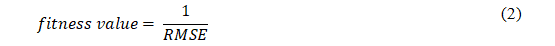2.4. Long-Short Term Memory (LSTM)

The LSTM machine learning method is based on the Recurrent Neural Network (RNN). RNN is a machine learning algorithm that uses repeated connections in each hidden layer unit that are interconnected at varying moments . LSTM was created to address the vanishing gradient problem in RNN . Unlike RNN, LSTM may hold long-term temporal dependence information and map between input and output data . The LSTM architecture is made up of a forget gate, an input gate, a cell state, and an output gate. The forget gate is the sigmoid layer that controls whether information from the cell state should be forgotten or replaced. The forget gate is determined by Equation 3.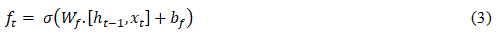Forget gate , as shown in Equation 3, is the sigmoid layer that is calculated from the product of weight matrixes forget gate  and  which is the output value at a time point and input value  which is then added to bias matrixes forget gate . For each number in the cell state, the forget gate produces a number between 0 and 1. What new information should be stored in the cell state is determined by the input gate. Equation 4 may be used to compute the input gate.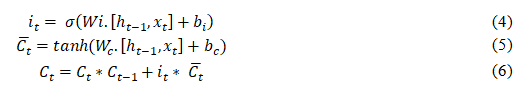Equation 4 is the equation used for the input gate which chooses which new information should be saved in the cell state. The candidate cell state that will be stored at time t is represented by Equation 5. Equation 6 is a formula to calculate the cell state at a given point in time t, by considering whether it is necessary to forget the previous cell state and what to consider in the cell state at the current time . The value selected by the sigmoid gate, as illustrated in Equation 7, is the output value.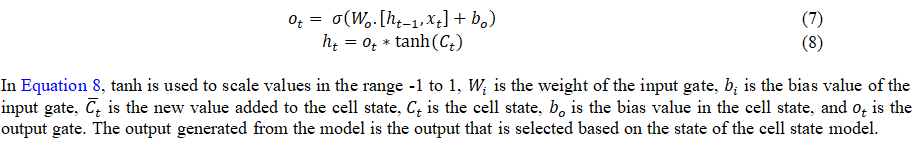2.5. Symbiotic Organism Search (SOS)

The symbiotic organism search (SOS) algorithm models the symbiotic relationships used by organisms to thrive in the environment . SOS, like other population-based metaheuristic algorithms, employs the population as a candidate solution in the search space to identify the best global answer. The purpose of metaheuristic algorithms is to solve optimization issues using computational intelligence paradigms . Since SOS has only one parameter, it can avoid parameter determination problems, which yield suboptimal solutions. SOS, in addition to being used to solve issues with a specific intent, may also be used to solve problems with many objectives . The SOS process begins with the selection of the starting population, known as the ecosystem. An initial ecosystem is a collection of creatures formed at random in the search space. Each creature symbolizes a potential solution to the situation at hand. Each creature in the ecosystem is assigned a fitness value that corresponds to the objective value of the problem to be addressed. Following the setup of the ecosystem, each creature goes through three processes: mutualism, commensalism, and parasitism, which imitate the biological interactions between two species in the ecosystem.

2.5.1. Mutualism Phase

During the mutualism phase, each organism engages in a mutually beneficial relationship to increase the benefits of living in the ecosystem. The mutualistic symbiosis between organisms Xi and Xj is used to compute new candidates for organisms Xi and Xj, as illustrated in Equations 9 and 10.As shown in Equation 9, Xinew is a new organism from Xi, where α is a vector with random values ranging from 0 to 1, Xbest is the best organism, Mutual Vector is computed using Equation 11, and Benefit Factor (BF1) is a random value ranging from 1 to 2. Xjnew, like the new organism Xinew, is a new organism derived from Xj using Equation 11. If Xinew has a higher fitness value than Xi, a new organism will replace an old one, and vice versa. This is likewise valid for Xjnew and Xj.

2.5.2. Commensalism Phase

In the commensalism phase, one organism benefits while the other does not. Organism Xj is depicted as an organism that will thrive or suffer as a result of its interaction with organism Xi. Based on the commensalism symbiosis between organisms Xi and Xj, a new organism Xinew is calculated as in Equation 12.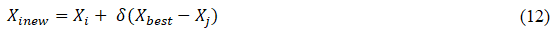As indicated in Equation 12, the new creature Xinew is created from the old organism Xi, which is computed based on a random selection of organisms Xj as the organisms that interact with organism Xi, where Xbest is the best organism and is a random value between -1 and 1.

2.5.3. Parasitism Phase

The parasitism phase describes the circumstance in which one organism thrives while harming another in the ecosystem. A Parasite Vector artificial parasite is a value chosen at random from the search space dimension. In the ecosystem, Parasite Vector will attempt to replace another organism, Xj. Darwin's theory of evolution states that "only the strongest creatures win." If Parasite Vector is superior, it will eliminate organisms Xj and take their place. Otherwise, if Xj possesses parasite immunity, Parasite Vector will be unable to exist in the environment.

2.6. Hybrid Model SOS – LSTM

Following the parameter initialization procedure, an ecosystem is constructed using a randomly selected combination of each hyperparameter and the fitness value as the best organism's initial. Two parameters will enter the mutualism, commensalism, and parasitism phases. SOS simulates the interplay of organisms' connections in order for them to survive in the environment. The three phases of mutualism, commensalism, and parasitism simulate the biological interactions between two organisms in an ecosystem.

Figure 3 depicts a flowchart of the three steps of SOS used to identify hyperparameter tuning on the LSTM model. The ecosystem will be set up at the start, and the best candidate will be chosen. Because the phenotypic of each creature is formed via a randomization process at this early stage, the ecosystem will consist of multiple species, each with a different phenotype. According to the hyperparameters that will be tuned, each organism will have six phenotypes. Following optimization, the model performance of each organism will be measured using a specified fitness value.

The next step is to select the best organism based on the greatest fitness value. For each step that is completed, the best organisms will be compared. Following the selection of the best organism, two separate organisms will be picked at random to start the mutualism phase.

Two new organisms will be calculated during the mutualism phase based on the benefit factor, mutual vector, and best organism. Mutualisms alter the proposed solution by calculating the difference between the two organisms' best and average performance (mutual vector). Because these two new species will have different phenotypes than the prior organisms, their model performance and fitness value will be revised at this point. If the new organism outperforms the old one, the new organism will take its position.

SOS uses the commensalism phase to compute the difference between alternative solutions. SOS exploits and optimizes other organisms similar to the best solution by using the best solution or the best organism as a reference point. This helps to enhance the speed of convergence on the task at hand, which in this case is determining the ideal hyperparameter for the LSTM.

During the parasitism phase, a mutation operator is developed and exploited in SOS. By selecting at random, the mutation vector or parasite vector will compete with other organisms. If this parasite vector outperforms the designated organism, the parasite vector will take its place. After going through the parasitism phase, the termination requirements for finding a solution will be evaluated again. A predefined number of iterations is the termination constraint to obtain this solution. If the termination requirements are not reached, the process will restart in search of the best creatures in the new environment, with each organism passing through the phases of mutualism, commensalism, and parasitism.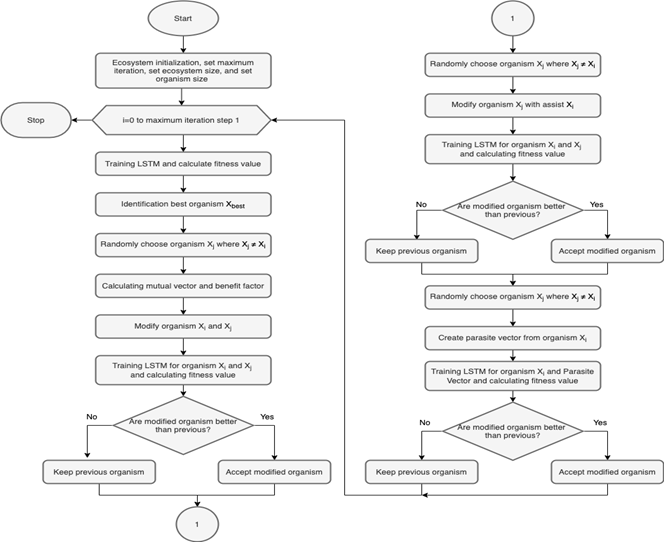Figure 3.Flow chart of hybrid SOS-LSTM model.

# 3. Results and Discussion

3.1. Dataset

The dataset utilized in this experiment consists of daily stock price data from 1990 to 2021. It contains statistics from the Indonesia Composite Index on stock prices. The dataset was obtained from the Yahoo Finance website. There are various variables in the dataset, including closing price, opening price, highest price, lowest price, and volume transactions. The closing price variable will be predicted by the machine learning model. Table 2 summarizes the statistical data from April 1990 to March 2021.

Table 2.Dataset description.
 Features Min Max Mean Standard Deviation Open 223.249 6687.231 2286.151 2117.722 High 223.249 6693.466 2299.109 2127.846 Low 223.249 6634.888 2271.446 2105.957 Close 223.249 6689.287 2286.319 2117.078 Volume 0.000 9788202500.000 34528049.212 211424174.102
3.2. Data Pre-Processing

The dataset then moves on to the data pre-processing step. After inspecting the dataset's statistical information, we discovered that it has many null values over multiple rows. Backward fill is the selected imputation approach. Backward fill will fill null values with the value of the closing price in the following observation. After filling in the null values, the subsequent step is scaling. The scaling method utilized is MinMaxScaler, which replaces the lowest and highest values of a feature with -1 and 1. The dataset is then divided into three sets: the training set, the validation set, and the test set. The proportions of the dataset used in the training set, validation set, and test set are 80%, 10%, and 10%, respectively. The goal of partitioning this dataset is to keep the machine learning model from overfitting. The validation result is used for hyperparameter tuning, thus it does not induce bias in the training model. The test set is used to compare and measure hybrid models SOS-LSTM, GA-LSTM, and PSO-LSTM.

3.3. Hyperparameters   Several hyperparameters in this experiment are tuned using a metaheuristic approach. As indicated in Table 3, the six hyperparameters have various value ranges. The metaheuristic method trains each hyperparameter using the same sliding window with a 20-day latency. The hyperparameters are given multiple lists, therefore the metaheuristic algorithm's search space is dependent on the value of each element in the list, ranging from 0 to 9. Each entry of the hyperparameters list has ten items. Using RMSE, the metaheuristics algorithm determines the optimal fitness value for each possible combination of hyperparameters in Table 3. The goal of each hybrid model is to minimize the RMSE value in each iteration.

Table 3. List of optimized hyperparameters.
 Hyperparameter Values Number of neurons [16, 32, 48, 64, 80, 96, 112, 128, 144, 160] Number of hidden layers [2, 4, 6, 8, 10, 12, 14, 16, 18, 20] Learning rate [0.01, 0.02, 0.03, 0.04, 0.05, 0.06, 0.07, 0.08, 0.09, 0.1] Optimizers [Adam, RMSprop, Adadelta] Dropout rate [0, 0.1, 0.2, 0.3, 0.4, 0.5, 0.6, 0.7, 0.8, 1] Number of epochs [10, 20, 30, 40, 50, 60, 70, 80, 90, 100]
3.4. Parameter Settings

Because CUDA-enabled GPUs can handle machine learning models with high time complexity and resource demand, the hybrid machine learning model LSTM with metaheuristic algorithms is computed using Compute Unified Device Architecture (CUDA). Nvidia's CUDA-enabled GPU is used in the CUDA platform. Each metaheuristic algorithm contains parameters that must be manually configured. The parameters of the Genetic Algorithm (GA) include population size n, mutation rate m, and crossover rate c. Aside from that, GA has additional settings that must be configured. We employ steady-state selection as the parent selection technique in GA and single-point crossover as the crossover type. The number of particles n, inertia weight w, cognitive coefficient c1, and social coefficient c2 are all separate parameters in Particle Swarm Optimization, whereas SOS requires just one parameter: the number of ecosystems n. Table 4 displays each value assigned by the metaheuristic method. The maximum iteration for any metaheuristic algorithm is 50 iterations.

Table 4.Parameter settings of the metaheuristic algorithms.
 GA PSO SOS n = 10 n = 10 n = 50 m = 0.15 w = 0.9 c = 0.7 c1 = 0.5 c2 = 0.3
3.5. Evaluation

Each hyperparameter combination was analyzed using Root Mean Square Error (RMSE) as the fitness value or major assessment measure. In addition, to choose the optimal hybrid machine learning model in the training, validation, and test sets, we employ Mean Absolute Error (MAE), Mean Absolute Percentage Error (MAPE), and R2 Score. Each metaheuristic method seeks to reduce the RMSE of the machine learning model. The purpose of RMSE is to calculate the standard deviation of the prediction error so that we can see how the predicted data spreads out and concentrates compared to the actual data. Equation 13 depicts the RMSE formula.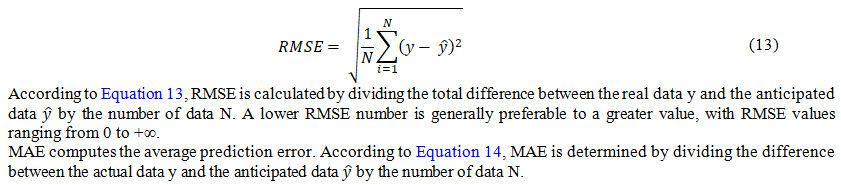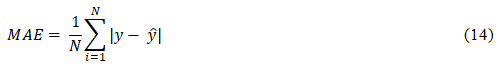MAPE is a metric for calculating the average percentage deviation error between predicted and actual data. MAPE is determined by dividing the difference between real data y and anticipated data  by actual data y and also by the number of data N. Equation 15 depicts the MAPE formula. A lower MAPE value is preferred to a higher value.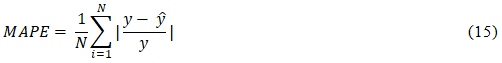The coefficient of determination, often known as the R2 score, is used to assess the goodness-of-fit of a linear regression model. The R2 score is derived by dividing the Mean Squared Error (MSE) of the machine learning model by the MSE of the base model, which is a horizontal straight line. The R2 score value range is -∞ to 1, with a greater R2 score value being preferable to a lower value. If the R2 score is close to 1, it indicates that the machine learning model matches the real data, and if it is close to -∞, it indicates that the machine learning model fits poorer than the horizontal straight line. Equation 16 shows how to compute the R2 score.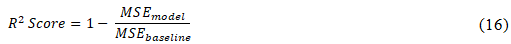RMSE, MAE, MAPE, and R2 scores are utilized as assessment measures in the training, validation, and test datasets. We compare assessment metrics from the training and validation datasets to see if underfitting or overfitting occurs. Overfitting occurs when prediction results substantially deteriorate invalidation. If the prediction result in training is lower than the other outcomes, underfitting occurs. The best hybrid machine learning model among GA-LSTM, PSO-LSTM, and SOS-LSTM is determined by the prediction results from the test dataset.

3.6. Experiment Result

﻿The purpose of hyperparameter tuning with a metaheuristic algorithm is to minimize the RMSE value in each iteration. RMSE is used to determine the fitness value; each iteration of the metaheuristic algorithm employs the RMSE from the validation dataset to eliminate bias in hyperparameter adjustment. Figure 4 depicts the convergence rate of the GA-LSTM, PSO-LSTM, and PSO-LSTM based on the RMSE value.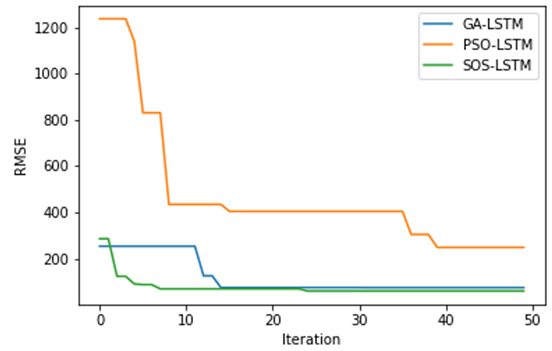Figure 4.Convergence of the GA-LSTM, PSO-LSTM, and SOS-LSTM.

SOS-LSTM outperformed GA-LSTM and PSO-LSTM in every iteration of the hybrid machine learning model. The SOS-LSTM convergence point is reached at the 26th iteration of 50 iterations, with an RMSE of 60.120. It outperforms GA-LSTM, which reached a convergence point at the 33rd iteration of 50 with an RMSE of 74.754, and PSO-LSTM, which reached a convergence point at the 41st iteration of 50 with an RMSE of 248.51.

Following the optimization of hyperparameter tuning in LSTM with a metaheuristic algorithm, the training process generates several outputs, including files containing the best hyperparameter configuration, machine learning model, evaluation metrics, best fitness value in each iteration, and prediction results from GA-LSTM, PSO-LSTM, and SOS-LSTM. The optimal hyperparameter configuration for GA-LSTM, PSO-LSTM, and SOS-LSTM can be seen in Table 5.

 Table 5. The best configuration of hyperparameters for GA-LSTM, PSO-LSTM, and SOS-LSTM.
 Hyperparameter GA-LSTM PSO-LSTM SOS-LSTM Number of neurons 160 48 32 Number of hidden layers 2 4 2 Learning rate 0.01 0.01 0.03 Optimizers Adam Adam Adam Dropout rate 0.2 0.3 0 Number of epochs 90 70 60

Each hybrid machine learning model has a unique set of hyperparameters. The number of neurons in SOS-LSTM is the lowest compared to the others, as seen in Table 5. Furthermore, the number of hidden layers or LSTM units in GA-LSTM and SOS-LSTM is the same (2 LSTM units), whereas PSO-LSTM has a greater number of hidden layers. GA-LSTM and PSO-LSTM both have a 0.01 learning rate, while SOS-LSTM has a higher learning rate of 0.03. Compared with RMSprop and Adadelta, all hybrid machine learning models consider Adam to be the superior optimizer. SOS-LSTM has the fewest epochs compared to the others. SOS-LSTM may offer the best assessment metrics with light architecture, as shown in Table 6.

Table 6.Evaluation metrics results in training and validation datasets.
 Methods Training Validation RMSE MAE MAPE R2 Score RMSE MAE MAPE R2 Score GA-LSTM 72.145 55.554 7.803% 0.997 74.754 57.731 1.105% 0.980 PSO-LSTM 151.413 118.669 17.998% 0.988 248.513 175.401 3.232% 0.779 SOS-LSTM 47.135 28.422 2.467% 0.998 60.120 44.866 0.880% 0.987

SOS-LSTM has the best assessment metrics for the training dataset compared to GA-LSTM and PSO-LSTM, despite achieving convergence point faster than GA-LSTM or PSO-LSTM. In the training stage, SOS-LSTM surpassed GA-LSTM with an RMSE of approximately 47.135 and PSO-LSTM with an RMSE of around 151.41. Figure 5 depicts a prediction result chart of GA-LSTM, PSO-LSTM, and SOS-LSTM that compares predictions with the actual data in the training stage.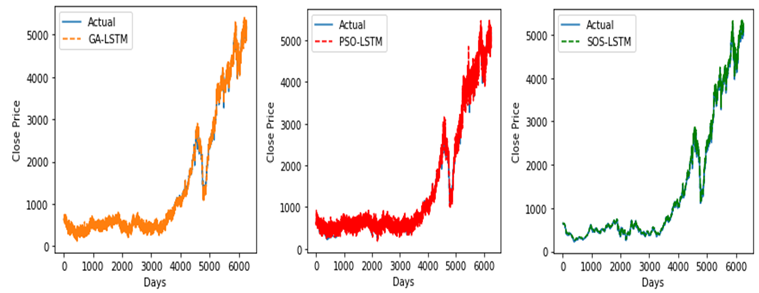Figure 5.The prediction results of the training data.

As shown in Figure 5, the SOS-LSTM prediction result when utilizing the training dataset has a strong fit with the actual data, with the best MAE, MAPE, and R2 scores attesting that the SOS-LSTM prediction result fits the real data. Figure 6 shows that PSO-LSTM is a poor match for the real data, although the R2 score of PSO-LSTM, approximately 0.988, is satisfactory. In PSO-LSTM, the high MAE, MAPE, and RMSE values indicate a larger prediction error. Figure 6 depicts the prediction results of GA-LSTM, PSO-LSTM, and SOS-LSTM compared to real data in the validation stage.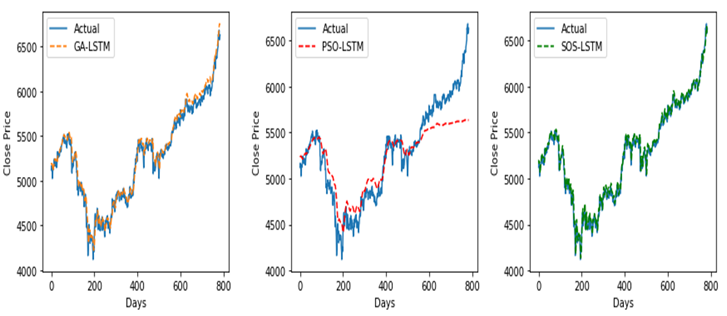Figure 6.Prediction results of the validation data.

The validation stage results demonstrate that SOS-LSTM and GA-LSTM do not exhibit a substantial decline in performance, indicating that there is no overfitting problem in the machine learning models SOS-LSTM and GA-LSTM. As shown in Table 6 and Figure 6, the prediction outcomes of PSO-LSTM decline substantially from an RMSE of around 151.41 in training to 248.51 in validation. The prediction result chart demonstrates that PSO-LSTM is a poor match for the real data. The low RMSE, MAE, MAPE, and R2 values confirm this. SOS-LSTM and GA-LSTM have comparable results, but SOS-LSTM has the highest RMSE, MAE, MAPE, and R2 scores. SOS-LSTM has an RMSE of approximately 60.120, an MAE of about 44.89, a MAPE of around 0.88 percent, and an R2 score of about 0.987. The final results of GA-LSTM, PSO-LSTM, and SOS-LSTM in the training stage are shown in Figure 7.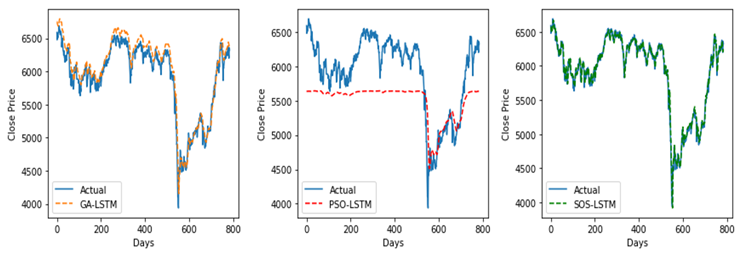Figure 7.Prediction result of test data.

The SOS-LSTM model outperformed both the GA-LSTM and the PSO-LSTM models. Figure 7 shows that SOS-LSTM is an ideal fit for the real data. This is confirmed by the greatest RMSE, MAE, MAPE, and R2 scores, as shown in Table 7. There is no substantial deterioration between validation and test evaluation metrics, indicating that the SOS-LSTM model creates a stable and resilient machine learning model that can fit unknown data. The RMSE of SOS-LSTM is approximately 78.799, the MAE is around 56.108, the MAPE is around 0.98 percent, and the R2 score is 0.98. Compared to GA-LSTM and PSO-LSTM, this is the best result.

Table 7. Comparative results of GA-LSTM, PSO-LSTM, and SOS-LSTM.
 Methods Test RMSE MAE MAPE R2 Score GA-LSTM 142.663 111.979 1.915% 0.935 PSO-LSTM 529.170 457.273 7.511% 0.111 SOS-LSTM 78.799 56.108 0.987% 0.980

# 4. Conclusion

This research implemented hyperparameter optimization of Long-Short Term Memory (LSTM), utilizing and comparing the metaheuristic algorithms Genetic Algorithm (GA), Particle Swarm Optimization (PSO), and Symbiotic Organism Search (SOS). In reality, data scientists seldom use metaheuristic algorithms to tune machine learning parameters, instead using Random Search, Grid Search, and Bayesian Optimization, which are prominent approaches to hyperparameter tuning. One of the reasons metaheuristic algorithms are rarely employed is that data scientists must select various parameters to obtain optimal results from metaheuristic algorithms. SOS offers benefits over other metaheuristic algorithms in that it has fewer parameters, which allows it to avoid parameter determination errors and provide solutions that are not optimal . Owing to this benefit, metaheuristic algorithms may be utilized as one of the most effective hyperparameter tuning approaches in practice.

The experimental findings reveal that SOS-LSTM achieves the best fitness value faster than GA-LSTM or PSO-LSTM. SOS-LSTM achieves convergence at the 26th iteration of 50 iterations with an RMSE of 60.120, which is better than GA-LSTM, which achieves convergence at the 33rd iteration of 50 with an RMSE of 74.754, and PSO-LSTM, which achieves convergence at the 41st iteration of 50 with an RMSE of 248.51. In the training, validation, and test stages, SOS-LSTM also generates a machine learning model that does not display overfitting and achieves a reasonable match with actual data. Finally, when compared to GA-LSTM and PSO-LSTM, SOS-LSTM has the best evaluation metrics, with an acquired RMSE of around 78.799, an MAE of around 56.108, a MAPE of around 0.98 percent, and an R2 score of 0.98. Based on these findings, Symbiotic Organism Search (SOS) could be utilized for hyperparameter tuning as it produces optimal and dependable solutions.

## References

  J. G. Agrawal, V. S. Chourasia, and A. K. Mittra, "State-of-the-art in stock prediction techniques," International Journal of Advanced Research Electronic Engineering Instruments, vol. 2, pp. 1360–1366, 2013.

  G. Chniti, H. Bakir, and H. Zaher, "E-commerce time series forecasting using LSTM neural network and support vector regression," in ACM International Conference Proceedings Series Policy, 2017, pp. 80–84.

  S. McNally, J. Roche, and S. Caton, "Predicting the price of bitcoin using machine learning," in Proceedings - 26th Euromicro International Conference on Parallel, Distrib. Network-Based Process PDP, 2018, pp. 339–343.

  H. Abbasimehr, M. Shabani, and M. Yousefi, "An optimized model using LSTM network for demand forecasting," Computers & Industrial Engineering, vol. 143, p. 106435, 2020.Available at: https://doi.org/10.1016/j.cie.2020.106435.

   N. Reimers and I. Gurevych, "Optimal hyperparameters for deep lstm-networks for sequence labeling tasks," arXiv preprint arXiv:1707.06799, vol. 1, pp. 1 - 34, 2017.Available at: https://doi.org/10.48550/arXiv.1707.06799.

  M. Hermans and B. Schrauwen, "Training and analyzing deep recurrent neural networks," in Proceedings of the 26th International Conference on Neural Information Processing Systems, 2013, pp. 190-198.

  M.-Y. Cheng and D. Prayogo, "Symbiotic organisms search: A new metaheuristic optimization algorithm," Computers & Structures, vol. 139, pp. 98-112, 2014.

   E. Celik, "A powerful variant of symbiotic organisms search algorithm for global optimization," Engineering Applications of Artificial Intelligence, vol. 87, p. 103294, 2020.Available at: https://doi.org/10.1016/j.cie.2020.106435.

  H. Wu, Y. Zhou, Q. Luo, and M. Basset, "Training feedforward neural networks using symbiotic, Corp," Computational Intelligence and Neuroscience, pp. 1–14, 2016.

 S. Siami-Namini, N. Tavakoli, and A. Siami Namin, "A comparison of ARIMA and LSTM in forecasting time series," in 17th IEEE International Conference on Machine Learning and Applications, ICMLA 2018, 2018.

   X. Qing and Y. Niu, "Hourly day-ahead solar irradiance prediction using weather forecasts by LSTM," Energy, vol. 148, pp. 461-468, 2018.Available at: https://doi.org/10.1016/j.energy.2018.01.177.

  H. Chung and K.-s. Shin, "Genetic algorithm-optimized long short-term memory network for stock market prediction," Sustainability, vol. 10, pp. 1-18, 2018.

  M. E. Abdual-Salam, H. M. Abdul-Kader, and W. F. Abdel-Wahed, "Comparative study between differential evolution and particle swarm optimization algorithms in training of feed-forward neural network for stock price prediction," presented at the INFOS2010 - 2010 7th International Conference on Informatics, 2010.

  C.-W. Tsai, "An effective WSN deployment algorithm via search economics," Computer Networks, vol. 101, pp. 178-191, 2016.

 A. S. Girsang, F. Lioexander, and D. Tanjung, "Stock price prediction using LSTM and search economics optimization," IAENG International Journal of Computer Science, vol. 47, pp. 758-764, 2020.

 M. Göçken, M. Özçalıcı, A. Boru, and A. T. Dosdoğru, "Integrating metaheuristics and artificial neural networks for improved stock price prediction," Expert Systems with Applications, vol. 44, pp. 320-331, 2016.Available at: https://doi.org/10.1016/j.eswa.2015.09.029.

  S. J. Nanda and N. Jonwal, "Robust nonlinear channel equalization using WNN trained by symbiotic organism search algorithm," Applied Soft Computing, vol. 57, pp. 197-209, 2017.Available at: https://doi.org/10.1016/j.asoc.2017.03.029.

  F. Nargesian, H. Samulowitz, U. Khurana, E. B. Khalil, and N. D. Turaga, "Learning feature engineering for classification.pdf," presented at the IJCAI International Joint Conferences on Artificial Intelligence, 2017

 G. H. T. Ribeiro, P. S. G. M. De Neto, G. D. C. Cavalcanti, and I. R. Tsang, "Lag selection for time series forecasting using Particle Swarm Optimization," in Proceedings of the International Joint Conference Neural Networks, 2011, pp. 2437–2444.

 P. E. Utgoff and D. J. Stracuzzi, "Many-layered learning," Neural Computation, vol. 14, pp. 2497-2529, 2002.Available at: https://doi.org/10.1162/08997660260293319.

 D. Srivastava, Y. Singh, and A. Sahoo, "Auto tuning of RNN Hyper-parameters using Cuckoo Search Algorithm," presented at the 12th International Conference on Contemporary Computing IC3, 2019.

 B. Yang, K. Yin, S. Lacasse, and Z. Liu, "Time series analysis and long short-term memory neural network to predict landslide displacement," Landslides, vol. 16, pp. 677-694, 2019.

 S. Hochreiter and J. Schmidhuber, "Long short-term memory," Neural Computation, vol. 9, pp. 1735-1780, 1997.

 K. Greff, R. K. Srivastava, J. Koutník, B. R. Steunebrink, and J. Schmidhuber, "LSTM: A search space odyssey," IEEE transactions on neural networks and learning systems, vol. 28, pp. 2222-2232, 2016.Available at: https://doi.org/10.1109/tnnls.2016.2582924.

 M. Abdel-Basset, L. Abdel-Fatah, and A. K. Sangaiah, "Metaheuristic algorithms: A comprehensive review," Computational Intelligence for Multimedia Big Data on the Cloud with Engineering Applications, vol. 1, pp. 185-231, 2018.Available at: https://doi.org/10.1016/B978-0-12-813314-9.00010-4.

 D.-H. Tran, M.-Y. Cheng, and D. Prayogo, "A novel Multiple Objective Symbiotic Organisms Search (MOSOS) for time–cost–labor utilization tradeoff problem," Knowledge-Based Systems, vol. 94, pp. 132-145, 2016.